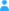Sign in

|

# Parameter square_​of_​geopotential_​height

## Properties

Name square_of_geopotential_height "square_of_X" means X*X. Geopotential is the sum of the specific gravitational potential energy relative to the geoid and the specific centripetal potential energy. Geopotential height is the geopotential divided by the standard acceleration due to gravity. It is numerically similar to the altitude (or geometric height) and not to the quantity with standard name height, which is relative to the surface. not specified not specified

## Source

The parameter was taken from the NetCDF CF Metadata Convention.

</